# NEET UG Physics Oscillations and Waves MCQs

NEET UG Physics Oscillations and Waves MCQs with answers available in Pdf for free download. The MCQ Questions for NEET Physics with answers have been prepared as per the latest NEET Physics syllabus, books and examination pattern. Multiple Choice Questions form important part of competitive exams and NEET exam and if practiced properly can help you to get higher rank. Refer to more topic wise NEET Physics Questions and also download more latest study material for all subjects and do free NEET Physics Mock Test

## Oscillations and Waves NEET Physics MCQ

NEET Physics students should refer to the following multiple-choice questions with answers for Oscillations and Waves in NEET. These MCQ questions with answers for NEET Physics will come in exams and help you to score good marks

### Oscillations and Waves MCQ Questions with Answers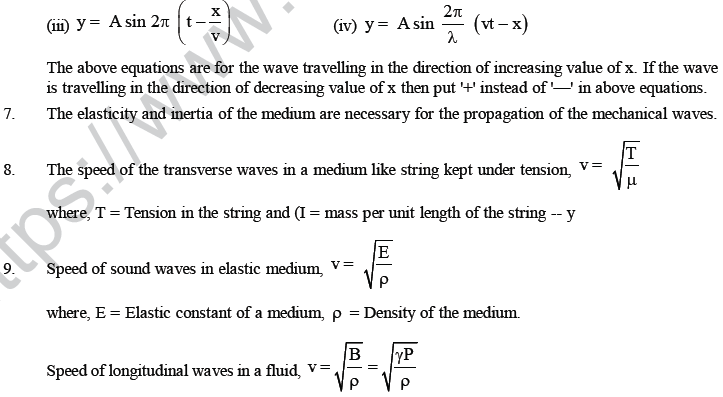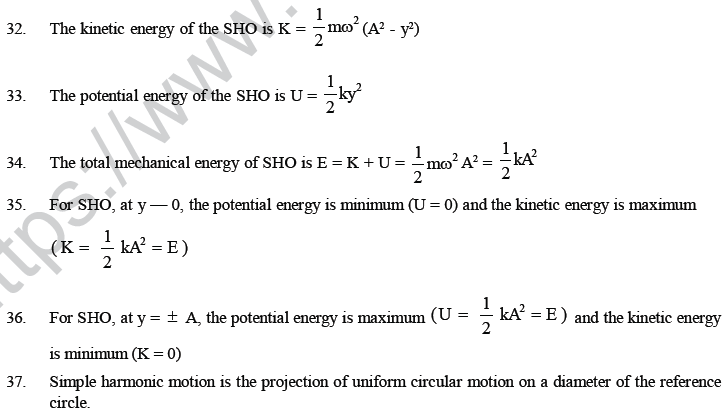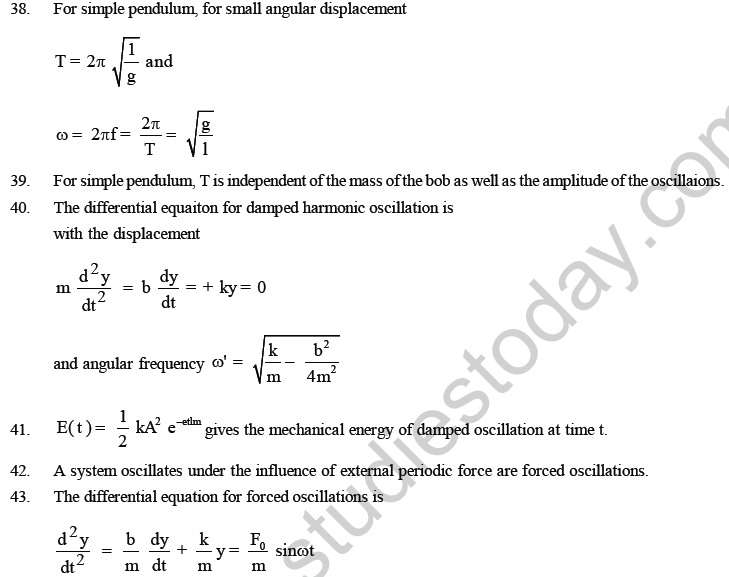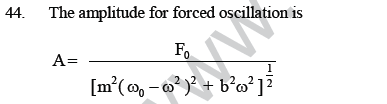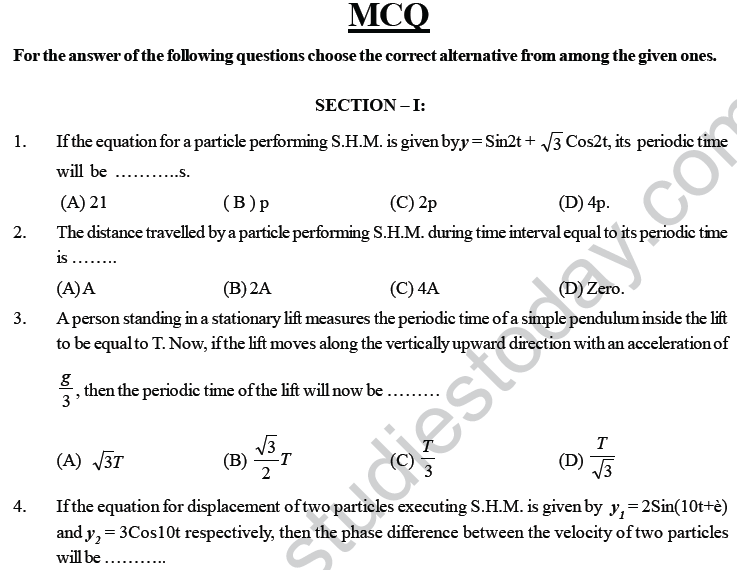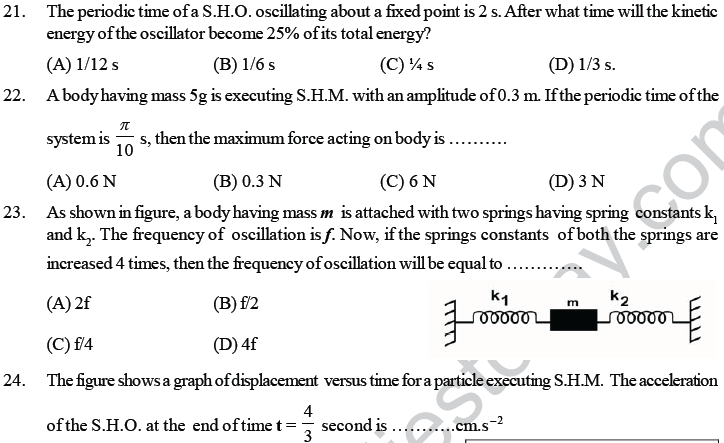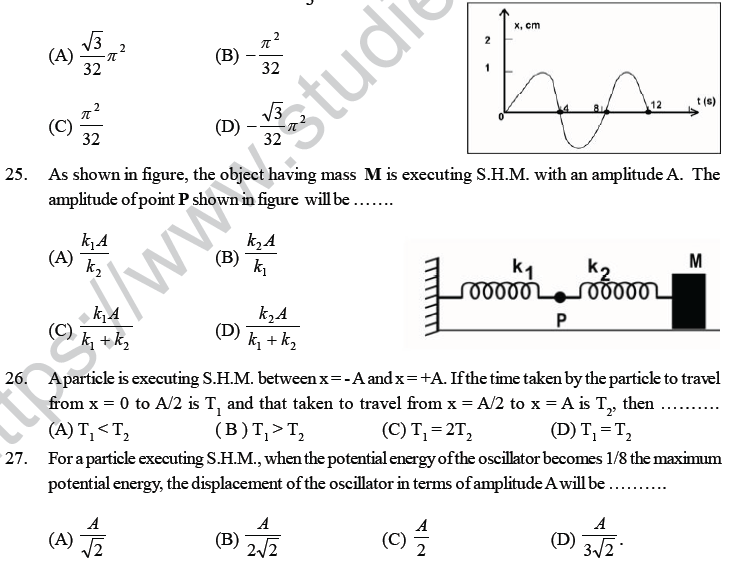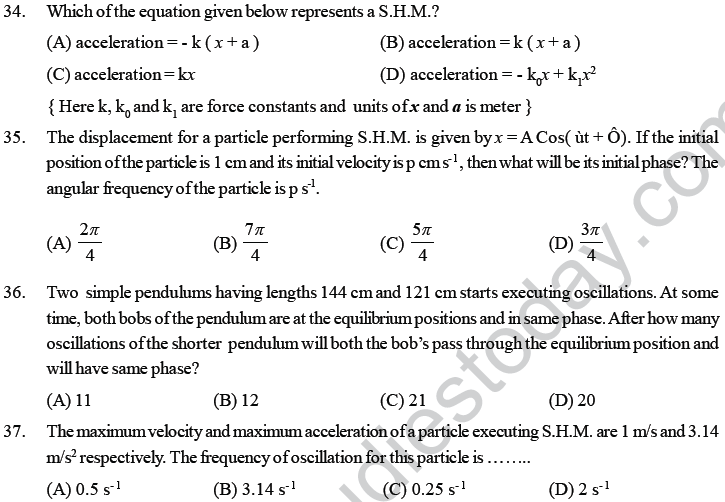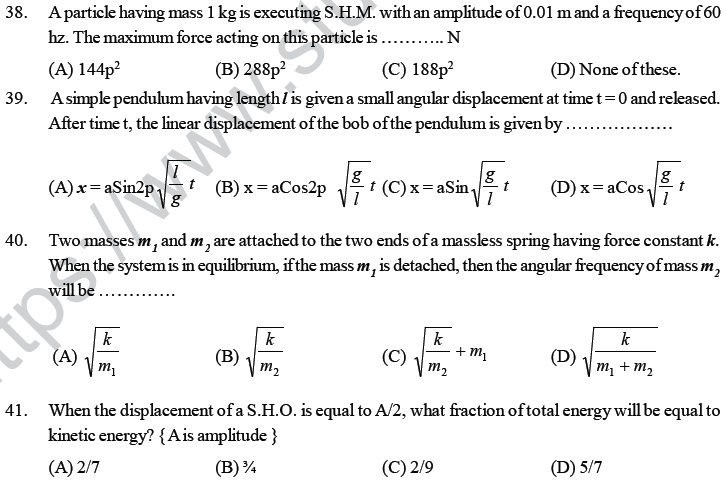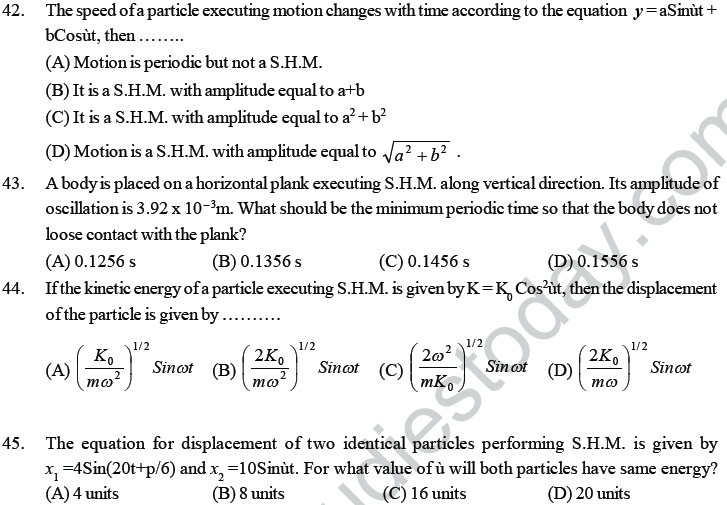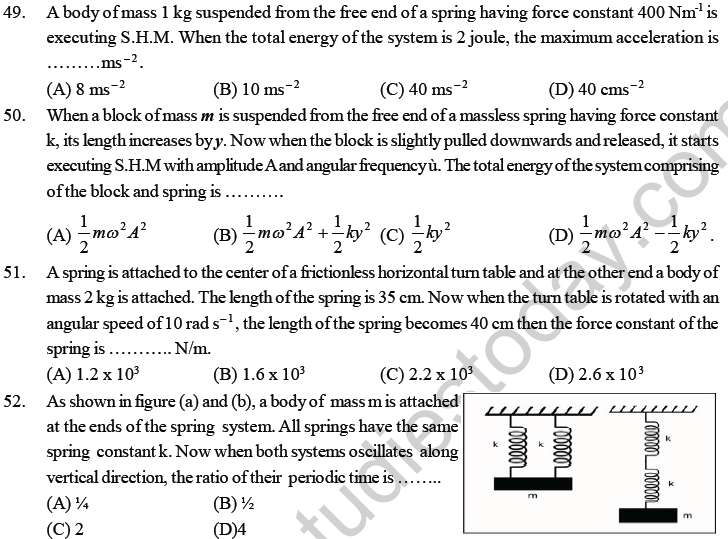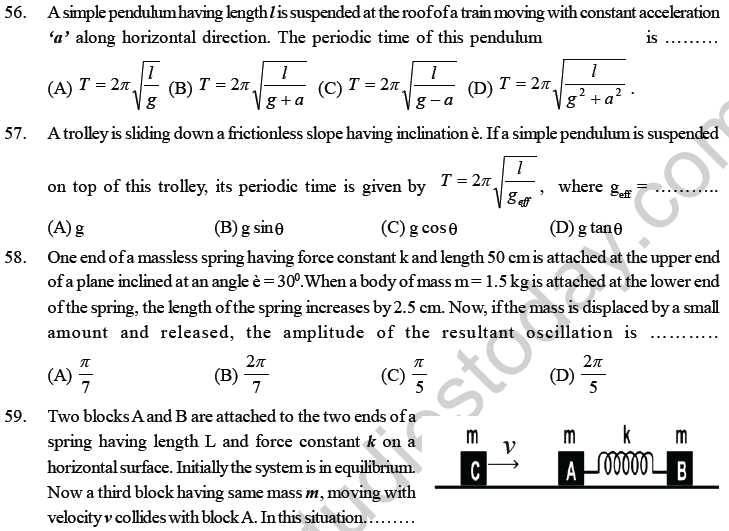SECTION : II
Assertion – Reason type questions :
Note:
For the following questions, statement as well as the reason(s) are given. Each questions has four options.
Select the correct option.
(a) Statement – 1 is true, statement- 2 is true; statement-2 is the correct explanation of statement – 1 .
(b) Statement – 1 is true, statement- 2 is true but statement-2 is not the correct explanation of statement – 1 .
(c) Statement – 1 is true, statement- 2 is false
(d) Statement – 1 is false, statement- 2 is true
(A) a (B) b (C) c (D) d

69. Statement – 1 : If a spring having spring constant k is divided into equal parts, then the spring constant of each part will be 2k.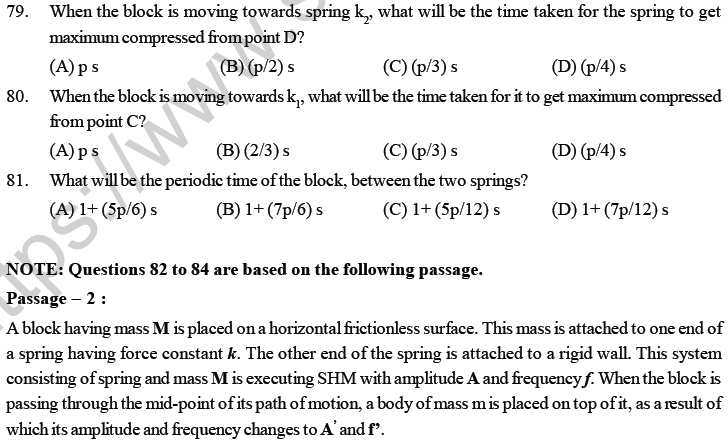NOTE: Questions 85 to 90 are based on the following passage.

Passage – 3:
The equation for displacement of a particle at time t is given by the equation y = 3Cos2t + 4Sin2t. .

85. The motion of the particle is ____
(A) Damped motion (B) Periodic motion (C) Rotational motion (D) S.H.M.

86. The periodic time of oscillation is ____
(A) 2 s (B) p s (C) (/2) s (D) 2p s

87. The amplitude of oscillation is ____ cm
(A) 1 (B) 3 (C) 5 (D) 7

88. The maximum acceleration of the particle is _____ cm / s2.
(A) 4 (B) 12 (C) 20 (D) 28

89. If the mass of the particle is 5 gm, then the total energy of the particle is ______ erg.
(A) 250 (B) 125 (C) 500 (D) 375

90. The frequency of the particle is ____ s-1 .
(A) (1/p) (B) p (C) (1/2p) (D) (p/2)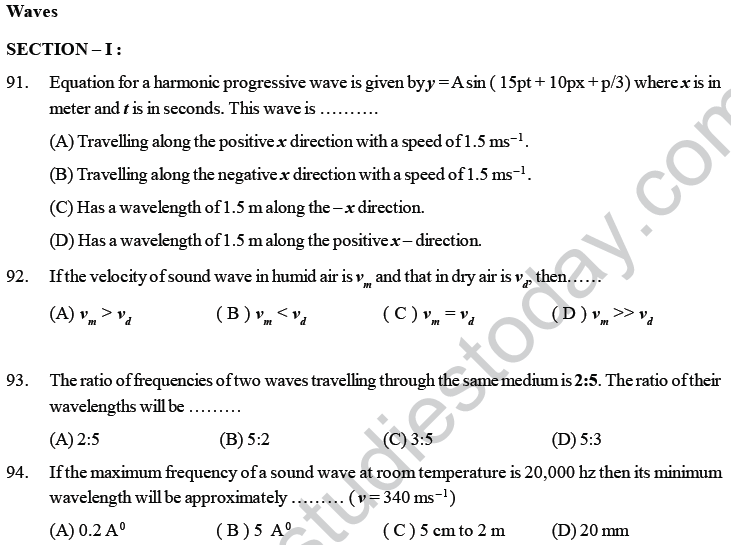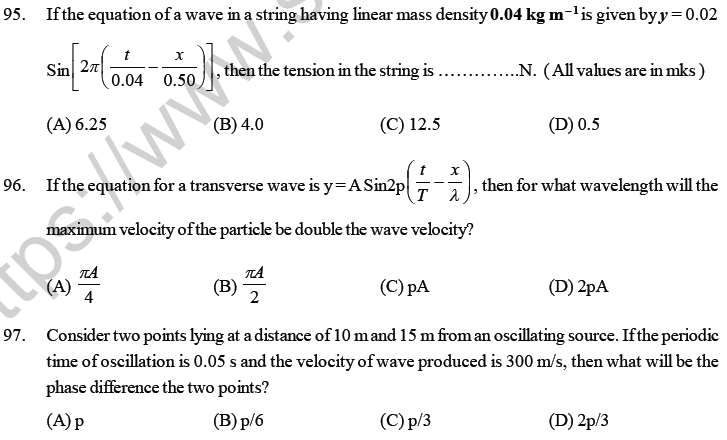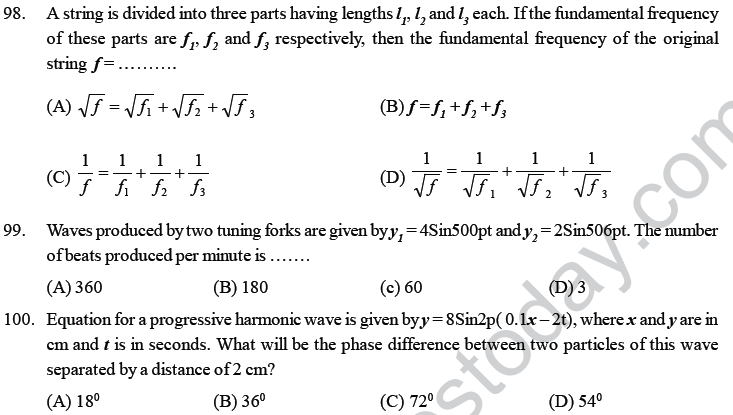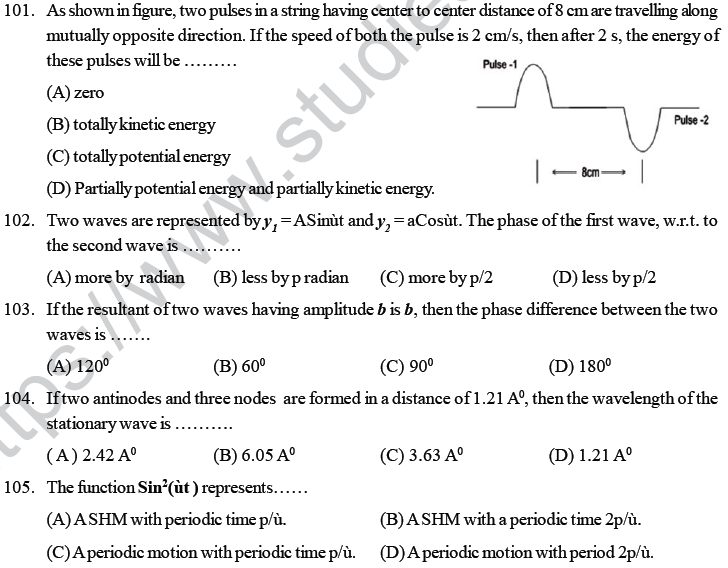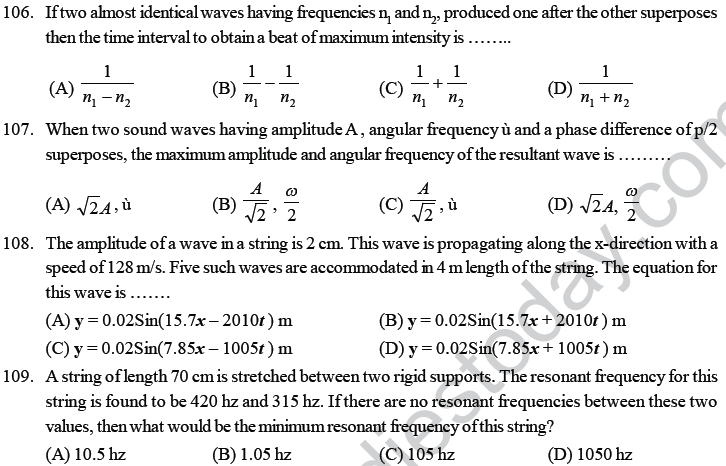114. Twenty four tuning forks are arranged in such a way that each fork produces 6 beats/s with the preceding fork. If the frequency of the last tuning fork is double than the first fork, then the frequency of the second tuning fork is ____
(A) 132 (B) 138 (C) 276 (D) 144

115. If two SHM’s are given by the equation y1 = 0.1 Sin(100pt + p/3) and y2 = 0.1 Cospt, then the phase difference between the velocity of particle 1 and 2 is ____
(A) p/6 (B) - p/3 (C) p/3 (D) - p/6

116. The wave number for a wave having wavelength 0.005 m is __ m –1 .
(A) 5 (B) 50 (C) 100 (D) 200

117. An listener is moving towards a stationary source of sound with a speed ¼ times the speed of sound.
What will be the percentage increase in the frequency of sound heard by the listener?
(A) 20% (B) 25% (C) 2.5% (D) 5%

118. When the resonance tube experiment, to measure speed of sound is performed in winter, the first harmonic is obtained for 16 cm length of air column. If the same experiment is performed in summer, the second harmonic is obtained for x length of air column. Then ___
(A) 32 > x > 16 (B) 16 > x (C) x > 48 (D) 48 > x > 32

119. What should be the speed of a source of sound moving towards a stationary listener, so that the frequency of sound heard by the listener is double the frequency of sound produced by the source?
{ Speed of sound wave is v }
(A) v ( B ) 2v (C) v/2 (D) v /4

120. A metal wire having linear mass density 10 g/m is passed over two supports separated by a distance of 1 m. The wire is kept in tension by suspending a 10 kg mass. The mid point of the wire passes through a magnetic field provided by magnets and an a.c. supply having frequency n is passed through the wire. If the wire starts vibrating with its resonant frequency, what is the frequency of a.c. supply?
(A) 50 hz (B) 100 hz (C) 200 hz (D) 25 hz124. A wave travelling along a string is described by y = 0.005Sin(40x – 2t) in SI units. The wavelength and frequency of the wave are _____
(A) (p/5) m ; 0.12 hz (B) (p/10) m ; 0.24 hz (C) (p/40) m ; 0.48 hz (D) (p/20) m ; 0.32 hz

125. Two sitar strings A and B playing the note “Dha” are slightly out of time and produce beats of frequency 5 hz. The tension of the string B is slightly increased and the beat frequency is found to decrease to 3 hz. What is the original frequency of B if the frequency of A is 427 hz?
(A) 432 (B) 422 (C) 437 (D) 417

126. A rocket is moving at a speed of 130 m/s towards a stationary target. While moving, it emits a wave of frequency 800 hz. Calculate the frequency of the sound as detected by the target. ( Speed of wave = 330 m/s)
(A) 1320 hz (B) 2540 hz (C) 1270 hz (D) 660 hz

127. Length of a steel wire is 11 m and its mass is 2.2 kg. What should be the tension in the wire so that the speed of a transverse wave in it is equal to the speed of sound in dry air at 20° C temperature?
(A) 2.31 x 104 N (B) 2.25 x 104 N (C) 2.06 x 104 N (D) 2.56 x 104 N

128. A wire stretched between two rigid supports vibrates with a frequency of 45 hz. If the mass of the wire is 3.5 x 10 –2 kg and its linear mass density is 4.0 x 10 - 2 kg/m, what will be the tension in the wire ?
(A) 212 N (B) 236 N (C) 248 N (D) 254 N

129. Tube A has both ends open while tube B has one end closed, otherwise they are identical. The ratio of fundamental frequency of tube A and B is ____
(A) 1:2 (B) 1:4 (C) 2:1 (D) 4:1

130. A tuning fork arrangement produces 4 beats/second with one fork of frequency 288 hz. A little wax is applied on the unknown fork and it then produces 2 beats/s. The frequency of the unknown fork is  _____ hz.
(A) 286 (B) 292 (C) 294 (D) 288

131. A wave y = aSin(ùt – kx ) on a string meets with another wave producing a node at x = 0. Then the equation of the unknown wave is _____
(A) y = a Sin(ùt + kx ) (B) y = - aSin( ùt+kx) (C) y = aSin(ùt – kx ) (D) y = - aSin(ùt – kx)

132. When temperature increases, the frequency of a tuning fork _____
(A) Increases (B) Decreases
(C) remains same (D) Increases or decreases depending on the material.

133. A tuning fork of known frequency 256 hz makes 5 beats per second with the vibrating string of a piano. The beats frequency decreases to 2 beats/s when the tension in the piano string is slightly increased. The frequency of the piano string before increase in the tension was ____ hz.
(A) 256 + 2 (B) 256 – 2 (C) 256 – 5 (D) 256 + 5.

134. An observer moves towards a stationary source of sound with a velocity one – fifth the velocity of sound. What is the percentage increase in the apparent frequency?
(A) 5% (B) 20% (C) zero (D) 0.5%

135. The speed of sound in Oxygen ( O2) at a certain temperature is 460 m/s. The speed of sound in helium at the same temperature will be _____ ms- 1 . (Assume both gases to be ideal)
(A) 330 (B) 460 (C) 5002 (D) None of these

136. In a longitudinal wave, pressure variation and displacement variation are………
(A) In phase (B) 90° out of phase (C) 45° out of phase (D) 180° out of phase

137. A tuning fork of frequency 480 hz produces 10 beats/s when sounded with a vibrating sonometer string. What must have been the frequency of the string if a slight increase in tension produces fewer beats per second than before?
(A) 480 (B) 490 hz (C) 460 hz (D) 470 hz

140. A string of linear density 0.2 kg/m is stretched with a force of 500 N. A transverse wave of length 4.0 m and amplitude 1/l meter is travelling along the string. The speed of the wave is ___m/s.
(A) 50 (B) 62.5 (C) 2500 (D) 12.5

141. Two wires made up of same material are of equal lengths but their radii are in the ratio 1:2. On stretching each of these two strings by the same tension, the ratio between their fundamental frequency is ____
(A) 1:2 (B) 2:1 (C) 1:4 (D) 4:1

142. The tension in a wire is decreased by 19%, then the percentage decrease in frequency will be ____
(A) 19% (B) 10% (C) 0.19% (D) None of these

143. An open organ pipe has fundamental frequency 100 hz. What frequency will be produced if its one end is closed?
(A) 100, 200, 300,____ (B) 50, 150, 250 ____
(C) 50, 100, 200, 300___ (D) 50, 100, 150, 200,____

144. A closed organ pipe has fundamental frequency 100 hz. What frequencies will be produced if its other end is also opened?
(A) 200, 400, 600, 800,….. (B) 200, 300, 400, 500, …..
(C) 100, 300, 500, 700,…… (D) 100, 200, 300, 400, …….

145. A column of air of length 50 cm resonates with a stretched string of length 40 cm. The length of the same air column which will resonate with 60 cm of the same string at the same tension is ____
(A) 100 cm (B) 75 cm (C) 50 cm (D) 25 cm

146. Two forks A and B when sounded together produce 4 beats/s. The fork A is in unison with 30 cm length of a sonometer wire and B is in unison with 25 cm length of the same wire at the same tension.
The frequencies of the fork are ____
(A) 24 hz, 28 hz (B) 20 hz, 24 hz (C) 16 hz , 20 hz (D) 26 hz, 30 hz

147. A tuning fork of frequency 200 hz is in unison with a sonometer wire. The number of beats heard per second when the tension is increased by 1 % is ___
(A) 1 (B) 2 (C) 4 (D) 0.5

148. A bus is moving with a velocity of 5 m/s towards a huge wall. The driver sounds a horn of frequency 165 hz. If the speed of sound in air is 335 m/s, the number of beats heard per second by the passengers in the bus will be ____
(A) 3 (B) 4 (C) 5 (D) 6

149. A vehicle with a horn of frequency n is moving with a velocity of 30 m/s in a direction perpendicular to the straight line joining the observer and the vehicle. The observer perceives the sound to have a
frequency ( n + n1 ). If the sound velocity in air is 300 m/s, then ___
(A) n1 = 10n (B) n1 = 0 (C) n1 = 0.1 n (D) n1 = - 0.1n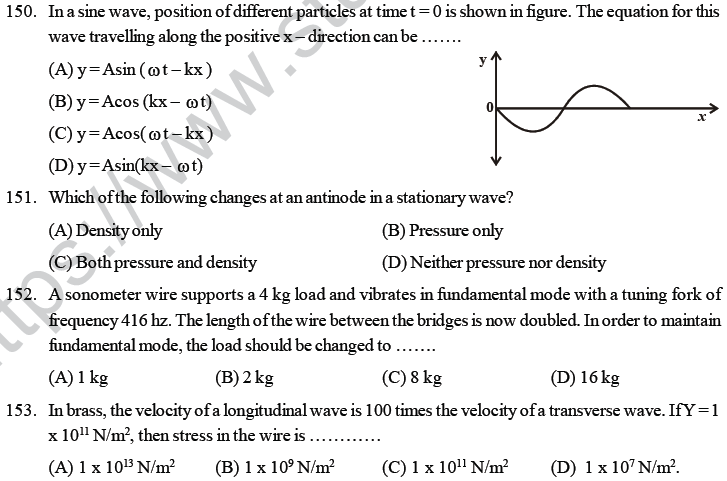157. A cylindrical tube open at both ends has a fundamental frequency f in air. The tube is dipped vertically in water so that half of it is in water. The fundamental frequency of the air column is now ____
(A) f/2 (B) f (C) 3f/4 (D) 2f

158. Three sound waves of equal amplitudes have frequencies (v-1 ), v, ( v+1). They superpose to give beats. The number of beats produced per second will be ____
(A) 3 (B) 2 (C) 1 (D) 4

159. A wave travelling along the x-axis is described by the equation y(x,t) = 0.005Cos(áx - ât). If the wavelength and the time period of the wave are 0.08 m and 2.0 s respectively, then á and â in appropriate units are _____
(A) á = 12.50p , â= p/2.0 (B) á = 25p , â= p
(C) á = 0.08/p , â= 2.0/p (D) á = 0.04/p , â= 1.0/p

160. A wave travelling along a string is described by the equation y = ASin(ùt-kx). The maximum particle velocity is _____
(A) Aù (B) ù/k (C) dù/dk (D) x

161. A string is stretched between fixed points seperated by 75 cm. It is observed to have a resonant frequencies of 420 hz and 315 hz. There are other resonant frequencies between these two. Then the lowest frequency for this string is ____ hz.
(A) 1.05 (B) 1050 (C) 10.5 (D) 105

162. Two tuning forks P and Q when set vibrating gives 4 beats/second. If the prong of fork P is filed, the beats are reduced to 2/s. What is the frequency of P, if that of Q is 250 hz.?
(A) 246 hz (B) 250 hz (C) 254 hz (D) 252 hz

163. The length of a string tied across two rigid supports is 40 cm. The maximum wavelength of a stationary wave that can be produced in it is ____ cm.
(A) 20 (B) 40 (C) 80 (D) 120

164. A stationary wave of frequency 200 hz are formed in air. If the velocity of the wave is 360 m/s, the shortest distance between two antinodes is ____ m
(A) 1.8 (B) 3.6 (C) 0.9 (D) 0.45

165. A tuning fork produces 8 beats per second with both 80 cm and 70 cm of stretched wire of a sonometer. Frequency of the fork is ___ hz.
(A) 120 (B) 128 (C) 112 (D) 240

166. An open pipe is in resonance in 2nd harmonic with frequency f1. Now one end of the tube is closed and frequency is increased to f2, such that the resonance again occurs in the nth harmonic. Choose the correct option.
(A) n = 3, f2 = (3/4)f1   (B) n = 3, f2 = (5/4)f1      (C) n = 5, f2 = (5/4)f1      (D) n = 5, f2 = (3/4)f1

SECTION : II
Assertion – Reason type questions :
Note:
For the following questions, statement as well as the reason(s) are given. Each questions has four options.
Select the correct option.
(a) Statement – 1 is true, statement- 2 is true; statement-2 is the correct explanation of statement – 1 .
(b) Statement – 1 is true, statement- 2 is true but statement-2 is not the correct explanation of statement – 1 .
(c) Statement – 1 is true, statement- 2 is false
(d) Statement – 1 is false, statement- 2 is true

167. Statement – 1: Two waves moving in a uniform string having uniform tension cannot have different velocities.
Statement – 2 : Elastic and inertial properties of string are same for all waves in same string. Moreover speed of wave in a string depends on its elastic and inertial properties only.
(A) a (B) b (C) c (D) d

168. Statement – 1: When a sound source moves towards observer, then frequency of sound increases.
Statement – 2 : Wavelength of sound in a medium moving towards the observer decreases.
(A) a (B) b (C) c (D) d

169. Statement – 1: Newton’s equation for speed of sound was found wrong because he assumed the process to be isothermal.
Statement – 2 : When sound propagates, the compressions and rarefactions happen so rapidly that there is not enough time for heat to be distributed.
(A) a (B) b (C) c (D) d

170. Statement – 1 : When pressure in a gas changes, velocity of sound in gas may change.
Statement – 2 : Velocity of sound is directly proportional to square root of pressure.
(A) a (B) b (C) c (D) d

171. Statement – 1 : If wave enters from one medium to another medium then sum of amplitudes of reflected wave and transmitted wave is equal to the amplitude of incident wave.
Statement – 2 : If wave enters from one medium to another medium some part of energy is transmitted and rest of the energy is reflected back.
(A) a (B) b (C) c (D) d

SECTION – III
COMPREHENSION BASED QUESTIONS
NOTE: Questions 172 to 174 are based on the following passage.
Passage – 1
A string 25 cm long and having a mass of 2.5 g is under tension. A pipe closed at one end is 40 cm long.
When the string is set vibrating in its first overtone and the air in the pipe in its fundamental frequency, 8 beats per second is heard. It is observed that decreasing the tension in the string decreases the beat frequency. The speed of sound in air is 320 ms – 1 .

172. The frequency of the fundamental mode of the closed pipe is ____ hz
(A) 100 (B) 200 (C) 300 (D) 400

173. The frequency of the string vibrating in its 1st overtone is ___ hz
(A) 92 (B) 108 (C) 192 (D) 208.

174. The tension in the string is very nearly equal to ____
(A) 25 N (B) 27 N (C) 28 N (D) 30 N

NOTE: Questions 175 to 178 are based on the following passage.
Passage – 2
Standing waves are produced by the superposition of two waves y1 = 0.05Sin(3pt – 2x) and y2 = 0.05Sin(3pt + 2x) where x and y are in meters and t is in seconds.

175. The speed ( in ms –1 ) of each wave is ____
(A) 1.5 (B) 3.0 (C) 3p/2 (D) 3p

176. The distance ( in meters ) between two consecutive nodes is ____
(A) p/2 (B) p (C) 0.5 (D) 1.0

177. The amplitude of a particle at x = 0.5 m is ____
(A) 1.08 x 10 –1 m (B) 5.4 x 10 –2 m (C) (p/2) x 10 –1 m (D) p x 10 –1 m

178. The velocity ( in ms –1 ) of a particle at x = 0.25 m at t = 0.5 s is ___
(A) 0.1p (B) 0.3p (C) zero (D) 0.3

177. The amplitude of a particle at x = 0.5 m is ___
(A) 1.08 x 10 –1 m (B) 5.4 x 10 –2 m (C) (p/2) x 10 –1 m (D) p x 10 –1 m

178. The velocity ( in ms –1 ) of a particle at x = 0.25 m at t = 0.5 s is ___
(A) 0.1p (B) 0.3p (C) zero (D) 0.3

NOTE: Questions 179 to 181 are based on the following passage.

Passage – 3

When two sound waves travel in the same direction in a medium, the displacement of a particle located at x at time t is given by y1 = 0.05Cos(0.50px - 100pt ) & y2 = 0.05Cos ( 0.46px - 92pt ), where y1, y2 and x are in meter and t is in seconds.

179. What is the speed of sound in the medium?
(A) 332 m/s (B) 100 m/s (C) 92 m/s (D) 200 m/s

180. How many times per second does an observer hear the sound of maximum intensity?
(A) 4 (B) 8 (C) 12 (D) 16

181. At x = 0, how many times between t = 0 and t = 1 s does the resultant displacement become zero?
(A) 46 (B) 50 (C) 92 (D) 100

NOTE: Questions 182 to 183 are based on the following passage.
Passage – 4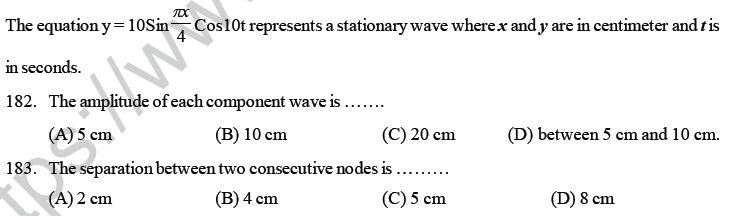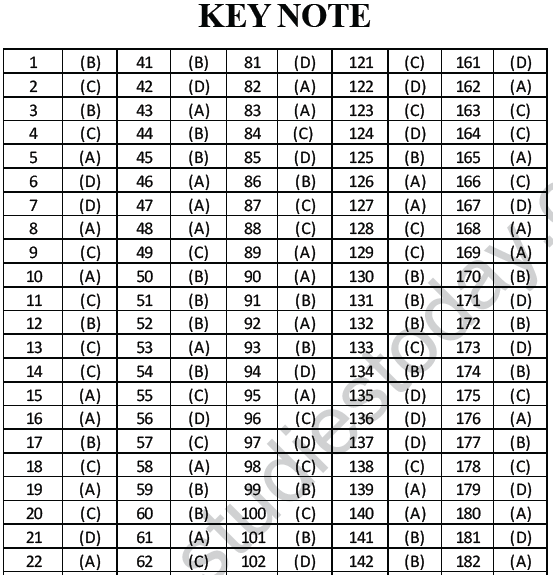## Tags:

Click for more Physics Study Material
 NEET UG Physics Properties of Liquid of Solid MCQs NEET UG Physics Kinetics MCQs NEET UG Physics Experiments MCQs NEET UG Physics Rational Motion MCQs NEET UG Physics Kinetic Theory of Gases MCQs NEET UG Physics Electrostatics MCQs NEET UG Physics Semiconductor Electronics MCQs NEET UG Physics Laws of Motion MCQs NEET UG Physics Electromagnetic Waves MCQs NEET UG Physics Thermodynamics MCQs NEET UG Physics Atom and Nucleus MCQs NEET UG Physics Magnetic Effects of Electric Current MCQs NEET UG Physics Electromagnetic Induction MCQs NEET UG Physics Work Energy MCQs NEET UG Physics Communication System MCQs NEET UG Physics Oscillations and Waves MCQs NEET UG Physics Gravitational MCQs NEET Physics Digital Electronics and Logic Gates MCQs NEET UG Physics Current Electricity MCQs NEET UG Physics Optics MCQs NEET UG Physics Kinetics and Measurement MCQs NEET UG Physics Dual Nature of Matter MCQs

## Latest NCERT & CBSE News

Read the latest news and announcements from NCERT and CBSE below. Important updates relating to your studies which will help you to keep yourself updated with latest happenings in school level education. Keep yourself updated with all latest news and also read articles from teachers which will help you to improve your studies, increase motivation level and promote faster learning

### Score well in Class 12 English Boards Exam

12th Board exams are an important part of students' lives. The marks obtained in the board exam decide the college in which one can study. In class 12 the syllabus of each and every subject increases vastly and it is difficult to cover up every point. In English also...

### CBSE Class 10 Revised Syllabus

Last year CBSE had to reduce the syllabus because of the pandemic situation but it was not very effective because there were no examinations. This year to avoid any confusion and conflict, CBSE has decided to reduce the syllabus into term 1 and term 2. 50 percent of...

### MCQ Question based CBSE examination

For 2021-22 CBSE has launched MCQ question-based examination for Term 1 & Term 2 board examinations. The entire syllabus has been divided into two parts each including 50% of the entire syllabus. To score well, students must practice as per the new CBSE term-wise...

### How To Solve Unseen Passages In English

Unseen passages may contain one or many paragraphs. This is one of the important yet easy parts for a student to get marks. Students should thoroughly study and understand the passage to answer the related questions. The unseen passages are there just to test the...

×